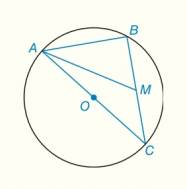Chapter 6.CT, Problem 9CTElementary Geometry For College St...

7th Edition
Alexander + 2 others
ISBN: 9781337614085

Solutions

Chapter
SectionElementary Geometry For College St...

7th Edition
Alexander + 2 others
ISBN: 9781337614085
Textbook Problem

In ⊙ O with diameter A C ¯   , O C = 5 and A B = 6   . If M is the midpoint of B C ¯ , find A M .To determine

To find:

AM of the circle O

Explanation

Given:

O with diameter AC¯, OC=5 and AB=6.

M is the midpoint of BC¯.

Theorem used:

An angle inscribed in a semicircle is a right angle”.

Calculation:

Consider,

Here OC is the radius of the circle given by OC=5.

Thus it is clear that the diameter AC=2(OC)=2(5)=10.

From the theorem it is clear that “an angle inscribed in a semicircle is a right angle”.

Here AC¯ formed a semicircle by B intercepts with the diameter.

Thus, ΔABC from a right angle.

Using Pythagoras theorem,

AC2=AB2+BC2102=62+BC210262=BC210036=BC264=BC2BC=8

Thus the length of BC is 8

Still sussing out bartleby?

Check out a sample textbook solution.

See a sample solution

The Solution to Your Study Problems

Bartleby provides explanations to thousands of textbook problems written by our experts, many with advanced degrees!

Get Started

In Exercises 7-28, perform the indicated operations and simplify each expression. 22. 2x(x+1)1/2(x+1)1/2x2

Applied Calculus for the Managerial, Life, and Social Sciences: A Brief Approach

Find the value of the sum. 27. i=04(2i+i2)

Single Variable Calculus: Early Transcendentals

The length of the curve given by x = 3t2 + 2, y = 2t3, is:

Study Guide for Stewart's Single Variable Calculus: Early Transcendentals, 8th